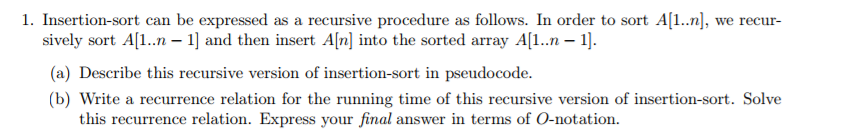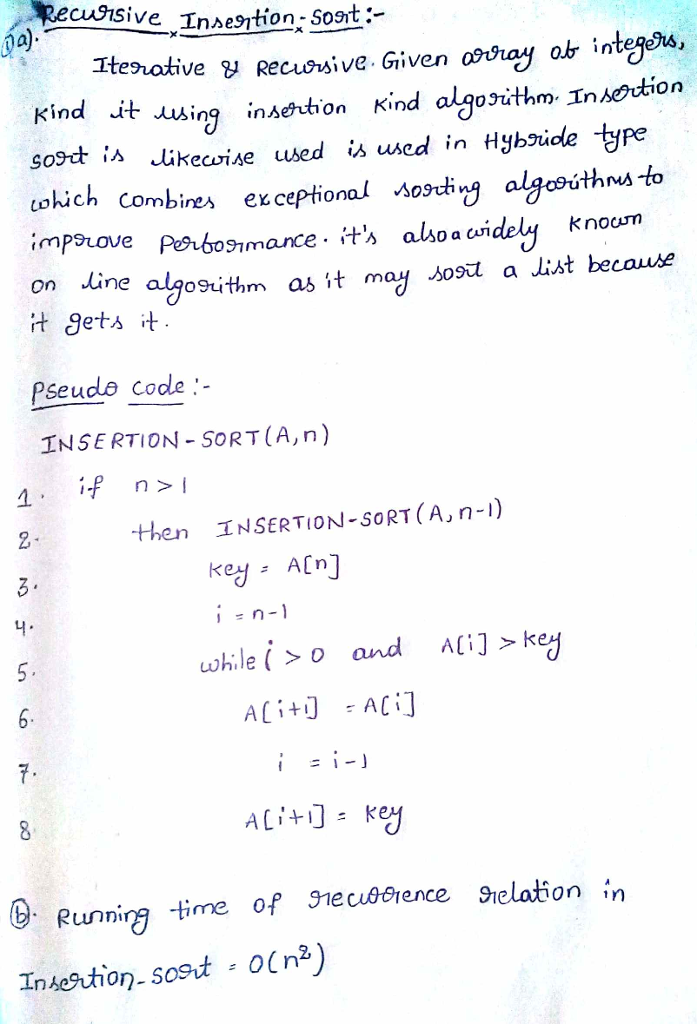# Homework Solution: Insertion-sort can be expressed as a recursive procedure as follows. In order to sort A[1..n], we recursively sort A[l..n-…Insertion-sort can be expressed as a recursive procedure as follows. In order to sort A[1..n], we recursively sort A[l..n-1] and then insert A[n] into the sorted array A[1..n-1]. (a) Describe this recursive version of insertion-sort in pseudocode. (b) Write a recurrence relation for the running time of this recursive version of insertion-sort. Solve this recurrence relation. Express your final answer in terms of O-notation.